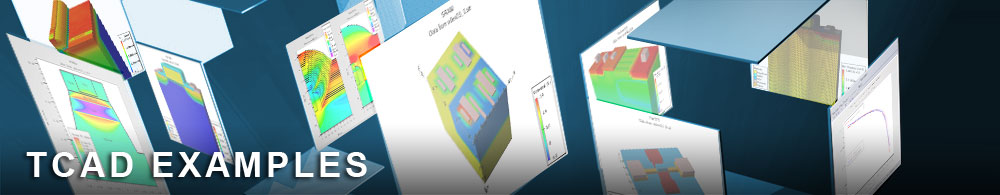Breakdown in SOI MOSFETs - Effect of Lattice Heating

## soiex06.in : Breakdown in SOI MOSFETs - Effect of Lattice Heating

Requires: S-Pisces/Giga
Minimum Versions: Atlas 5.28.1.R

This example compares Ids/Vds analyses of a short channel ultra-thin SOI transistor with Energy Balance (EB) and Nonisothermal Energy Balance Models (NEB). It shows:

• SOI structure formation using Atlas syntax
• Selection of energy balance and lattice heating models
• Selection of numerical methods for coupled solution technique
• Id/Vds simulation for Vgs=4.0V

The example file consists of two runs. The first simulates the device using energy balance models, the second using energy balance and lattice heating models combined.

Submicron devices should be simulated using the Energy Balance Model due to velocity overshoot, and nonlocal impact ionization effects, which could substantially influence device characteristics. For high current levels (high gate voltages) the thermal self-heating effects could also play an important role decreasing mobility and impact ionization rate. These effects are very pronounced in SOI transistors due to low heat conductivity of the oxide. This leads to the negative output conductance. This example demonstrates comparison of Ids/Vds curves obtained with Energy Balance and Nonisothermal Energy Balance Models.

The first Atlas run uses the Energy Balance Model: Poisson's equation, carrier continuity equations, and the energy balance equation for electrons are solved self-consistently.

The device is described in the first part of the input file. The SOI device is composed of a 0.05 micron layer of silicon on a 0.45 micron silicon dioxide substrate. The device has a 12 nm thick gate oxide and a gate length of 0.8 microns. Effective channel length is 0.5 microns.

After the device description, the material statement is used to assign an energy relaxation time for electrons. The models hcte.el statement is used to set the solution for electron energy balance. Other parameters on the model statement select a set of physical models for this simulation. In this case, these models are CONSRH and AUGER recombination, the conmob and fldmob mobility model and Band Gap Narrowing. The impact length.rel lrel.el=0.02 statement is used to assign the energy relaxation length for Selberherr model. The contact statement is used to assign the work function on the polysilicon gate.

The gate voltage is ramped to 4V. At this stage the combined algorithm is used: method gummel newton carr=2 . Thus if convergence is not reached in decoupled mode (gummel) the simulator will automatically switch to coupled mode (newton). Then the drain voltage is ramped. Again the combined algorithm is used for low drain biases and full newton scheme for higher drain biases. The method statement also defines that a two carrier solution is performed using carr=2 whereby Atlas will solve Poisson's equation for potential and the electron and hole continuity equations.

The second Atlas run uses Nonisothermal Energy Balance Model: Poisson's equation, carrier continuity equations, energy balance equation for electrons, and a lattice energy balance equation are solved self-consistently.

The same set of models is used, except that the solution of the lattice energy balance equation is activated using lat.temp parameter in the models statement.

In addition, thermal boundary conditions should be defined in this case. Thermal boundary conditions are defined in the thermcontact statement. A 300K temperature is specified at the thermcontact located along the bottom of the device. Thermal isolation conditions are assumed on all other surfaces.

Id/Vds curves from the two simulations can be compared in TonyPlot. Internal variables at the same bias point can also be compared using the saved solution files. Plots of impact ionization rate and electron temperature show the effect of the elevated temperature in the silicon film of these quantities.

To load and run this example, select the Load button in DeckBuild > Examples. This will copy the input file and any support files to your current working directory. Select the Run button in DeckBuild to execute the example.